home |  electronics |  toolbox |  science club |  tuxtalk |  photos |  e-cards |  online-shop

http://tuxgraphics.org/electronics## Programmable 8-character LCD module and digital voltmeter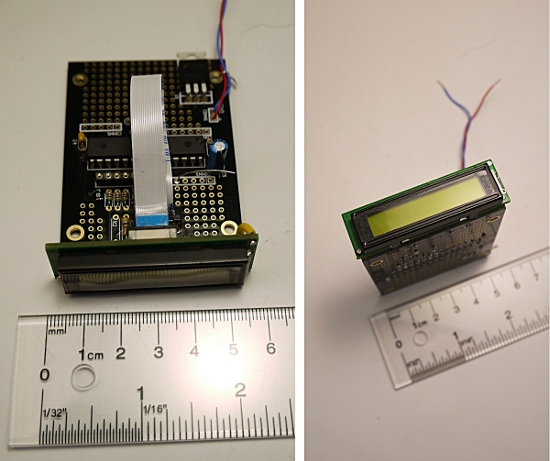Abstract:

Our 3 digit LED digital voltmeter module has been quite successful over the years. This new LCD module is basically an advanced version with a lot more capabilities. You can power it with the same voltage source that your signal is derived from. You can load your own code into this module but it has even some nice features for people who just need a voltmeter module and don't want to play with C-code.

_________________ _________________ _________________

## Signal and power from the same voltage source

Most hobby electronics store carry cheap and ready to use digital voltmeter modules (DVM) the problem with those is that they do usually require an independent power source to power the DVM-module. Many circuits are made such that you have one power supply and you would like to power your circuit and the voltmeter module from this one single power supply.

This is exactly how you can use our 8-character LCD module and it saves you a lot of headaches on the power supply side. The module can either be powered with exactly 5V DC (without the voltage regulator installed) or it can be powered with a DC voltage of 6.5V or more.

## A display that works in the dark and in full sun light

We have used an LCD display that has back lighting but is at the same time able to take advantage of any surrounding light sources. In other words you get a module that works well in the dark and in full sun light. The readability is very good.

## Space for your own sensor and components

This module is not just a display. It has as well a dot-matrix area where your can add your own components.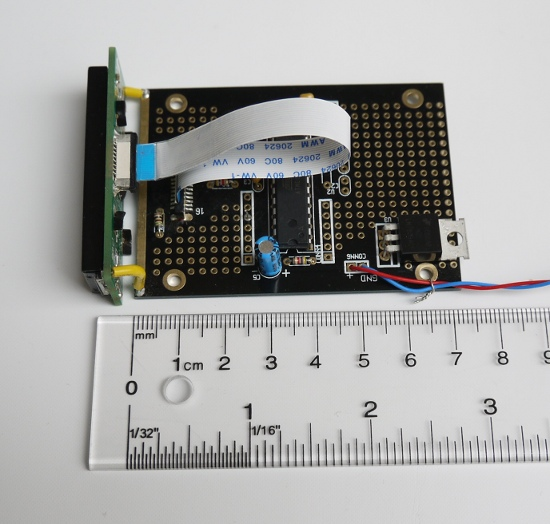The dot matrix field on the 8-character DVM module, space for your own components

## How can I program this 8 character display module?

The system has an atmega328p (or atmega328) chip and you can basically program it in any way you like and make it do anything that a microcontroller can do: Time events, read digital or analog signals, output digital signals, interact with the users.

The display is 8 characters long and fully alphanumeric. You can display letters, punctuation and numbers.

The possibilities are almost endless you just need to write the software for it. We provide some basic examples in the download section at the end of this article. You can take that code and use it to build your own application.

## A configurable DVM module: flexible with no need to write any software

You can write your own software for this module but it is optional. It comes with software loaded and this default software covers a wide range of applications.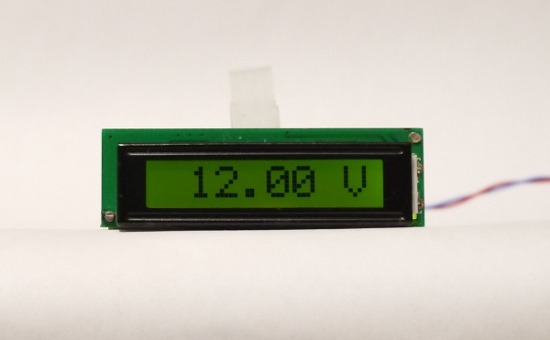The configurable DVM showing 12V with the unit Volt behind the numbers

We have noticed that a lot of user want to measure analog signals, run them through some linear formula and then display the result. All our "8 digit LCD modules" come now with software per-loaded that allows you to do such things without writing code. You just set a jumper wire and you enter a setup mode where you can enter the parameters for a linear equation.
```The equation you can configure is:

Display_value = Analog_to_digital_converter_output * M / D + A
```

M, D and A are configurable constants (M=multiply, D=divide, A=add or subtract). All math is done with integers and from left to right in the above equation. You can as well configure a parameter P which is the amount of digits behind the decimal point. In other words you can e.g set P=2 which will then display 10 as 0.10 and 100 as 1.00 etc... The "Analog_to_digital_converter_output" is an integer number that the analog to digital converter (ADC) provides and in this case it is a number between zero and 4092 (12 bit with oversampling).

It is as well possible to optionally configure a short two character string that will be shown behind the numbers in the display. This can be used to display a measurement unit such as "mA" or "'C".

## A configurable DVM module: Dual voltmeter

As of firmware version 1.1 this configurable digital voltmeter supports the measurement of two voltages. Thus you can measure two different signals: One analog signal on ADC0 and another one on pin ADC1.

This display can then be switched between the two channels:

Note that both analog channels (ADC0 and ADC1) have one common ground pin.

## A configurable DVM module: How to configure it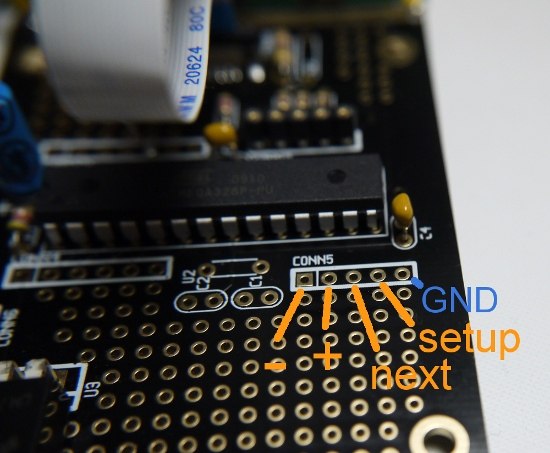A configurable DVM module, no need to write any C-code

There is no computer required to change the linear equation that has been programmed into this module. You just connect a temporary jumper wire from the "setup" pin to GND and your power cycle the module. The string "setup" appears in the display and your are in setup mode. By touching the "next" pin with a wire connected to GND you go through the different configuration steps as shown in the below diagram.
 ``` | V | setup pin -> GND at power on | module enters setup mode | | next pin -> GND | module shows raw analog digital converter readings. ADC0 with 12bit accuracy. The display shows "a0:" followed by numbers between 0 and 4092. | | next pin -> GND | Module shows "D:" and you can use the pins "+" and "-" to change the value for the divider. Touch "+" or "-" with a GND connected wire to step the value up or down and connect the wire for a longer time to fast scroll up or down. | | next pin -> GND | Module shows "M:" and you can use the pins "+" and "-" to change the value for the multiplier. The handling is the same as described above for "D:". | | next pin -> GND | Module shows "A:" and you can use the pins "+" and "-" to change the value for the add or subtract constant. The handling is the same as described above for "D:". | | next pin -> GND | Module shows "P:" and you can use the pins "+" and "-" to change the value for the amount of digits behind decimal point. The handling is the same as described above for "D:". | | next pin -> GND | Module shows "c0:none" and you can use the pins "+" and "-" to change the "none" into a letter (can be used to display the measurement unit). Possible letters are one of the following: ABCDEFGHIJKLMNOPQRSTUVWXYZ '%\$ abcdefghijklmnopqrstuvwxyz Note: programming c0 to none means not showing any units indepdent of what values are set for c1 and c2. You should program c0 to a single space character if you want a bit of space between the digits and the measurement unit. | | next pin -> GND | Module shows "c1:none" and you can use the pins "+" and "-" to change the "none" into a second letter (can be used to display the measurement unit). | | next pin -> GND | Module shows "c3:none" and you can use the pins "+" and "-" to change the "none" into a second letter (can be used to display the measurement unit). | | next pin -> GND | The values are stored permanently in EEPROM and the module shows "done" in the display. Power-cycle the module. ```

You can power off the module at any point in time to exist the setup. This is e.g useful if you don't want to change any setting and you just want to monitor the raw ADC0 or ADC1 readings.

To configure the formula for analog values read from ADC0 you leave pin PD0 open. To configure the formula for from ADC1 you connect PD0 to GND prior to entering the configuration mode. To use this as a full dual voltmeter with two formulas you would enter setup mode (configuration mode) twice.

## A configurable DVM module: How to dimension the hardware

The configurable DVM module allows you to measure anything that is linear in nature and display it in the desired unit. You could take an analog temperature sensor (which produces a temperature dependent voltage) and show the result on the display in 'C or 'F. You can use it to measure any voltage and display it in Volt or any current and display it in Ampere.

Note: Be careful with voltages above 48V, those can be dangerous without proper insulation.

To do this the following simple steps are needed:
1. Convert the DC input voltage to the right max. value. The ADC on the board works with voltages from 0 to 1.1V and whatever you measure needs to be electrically converted into that voltage range. If the max. expected voltage is higher tan 1.1V then a voltage divider (2 resistors) is required. If the max. expected voltage is much lower than 1.1V then an amplifier is required.
2. Convert the integer numbers from the ADC (0-4092, 4092 instead of 4096 because we use oversampling to achieve 12bit accuracy) into the physical units that you like to display (voltage, temperature, pressure, ...). If a sensor is used then this step might requires knowledge about the conversion that happens in the sensor (e.g temperature to voltage).
3. Display the measurement results.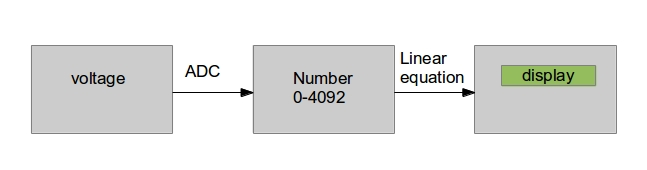The different conversion steps

## A configurable DVM module: dimension voltage divider or pre-amplifier

If you need to reduce the voltage (e.g measure voltages from 0-10V) then you will need to add a voltage divider. I suggest to add a capacitor to filter out any noise especially the 50Hz/60Hz "power-line hum" that surrounds us everywhere.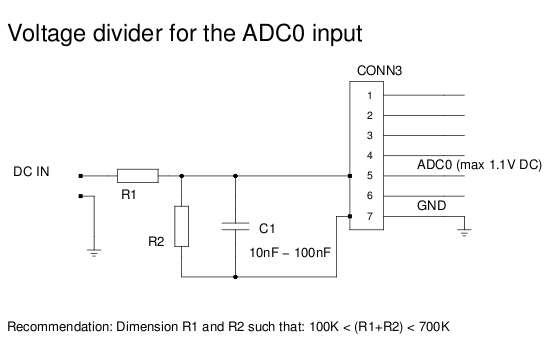Voltage divider and noise filter. Any capacitor between 10nF and 100nF will work for this noise filter.

We have created two javascript based calculators to help you find the right values for the resistors in the voltage divider. The first calculator works such that you just enter the max voltage that you would like to measure and the calculator suggests resistors based on the E12 resistor series: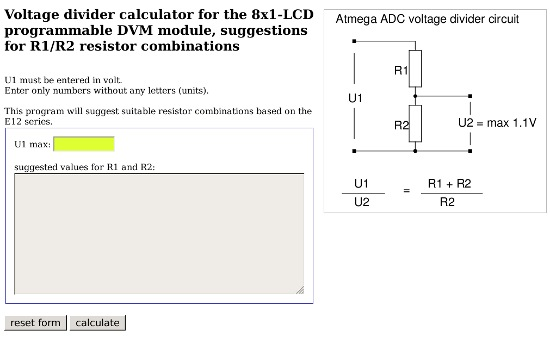Just enter the max voltage and the calculator computes suitable resistor values.
Click on the image to start the javascript based calculator.

The second calculator is more manual. You enter a resistor and the maximum voltage and the calculator computes the other resistor or you enter two resistor values and the calculator computes the maximum voltage that you can measure. This calculator is very useful if you want to use parallel resistors or two resistors in series to get in-between values which are not part of the E12 series.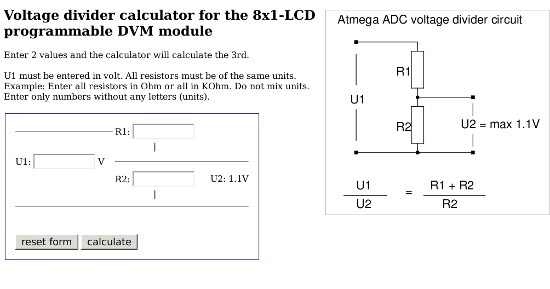A general voltage divider calculator.
Click on the image to start the javascript based calculator.

An amplifier is needed if your expected max. input voltage is much smaller than 1.1V. The 8-character LCD module works internally with 5V power and there is a voltage regulator on board that reduces any external supply voltage to 5V. You can use those five volts to power a small pre-amplifier circuit. This pre-amplifier can fit onto the dot-matrix field of the 8-character LCD module.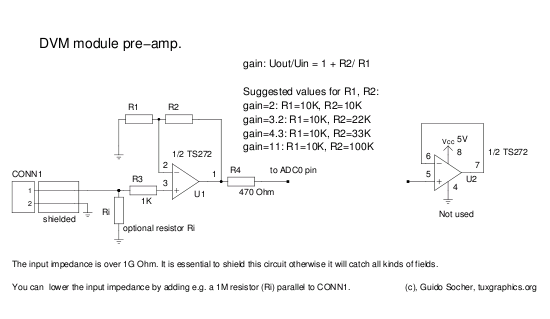A simple linear pre-amplifier that works with 5V DC.

## A configurable DVM module: configuring the linear equation

We have configured the hardware to provide the right signal level to the board and now we just have to configure the linear equation such that the display shows us whatever we want to measure in the right format.

The linear equation programmed into the configurable DVM module looks as follows and you can change the constants M,D and A as well as a parameter P that defines the amount of digits behind the decimal point:
```Display_value = Analog_to_digital_converter_output * M / D + A
```

The "Analog_to_digital_converter_output" is the input voltage divided by the division factor that the voltage divider adds (or multiplied by the gain of a possible pre-amp) times 4092/1.1V:
```Display_value = Vin/Rdiv * 4092/1.1V * M / D + A
```

Vin = voltage before the voltage divider
Rdiv = the factor by which the voltage divider reduces the voltage: Rdiv=(R1+R2)/R2

This formula can now be used to select suitable values for M, D and A. If there is a sensor involved then the conversion that this sensor does needs to be added as a factor in the equation (e.g 1V/4'C for A Temperature sensor, refer to the datasheet of your sensor).

## A configurable DVM module: A few real world examples

### First example: Measure voltages from 0-25V DC, show result with two digits behind the decimal point.

We add a suitable voltage divider that will convert 25V to a max of 1.1V (the max. voltage that the analog to digital converter takes):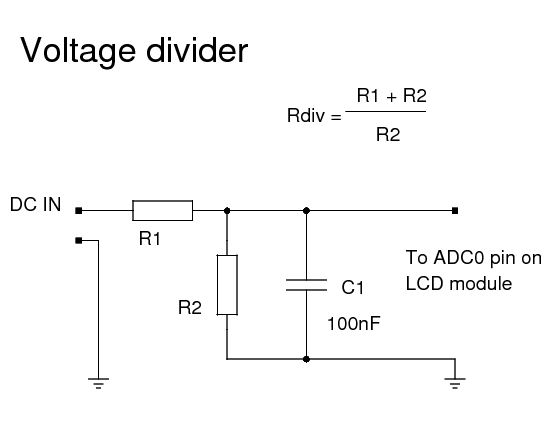Voltage divider

To do this you open the above mentioned javascript based calculator in your web browser. You enter "25" and you press "calculate". The calculator suggest ten different resistor combinations and you just choose.
```...
R1=180 KOhm, R2=8.2 KOhm, U1max=25.02V, resulting Ri=188.2 KOhm
R1=220 KOhm, R2=10 KOhm, U1max=25.07V, resulting Ri=230 KOhm
R1=270 KOhm, R2=12 KOhm, U1max=25.62V, resulting Ri=282 KOhm
...
```
For this example we will use: R1=220 KOhm, R2=10 KOhm.
The division factor is R1+R2/R2=23

We want to show the result with two digits behind the decimal point. In other words we want to show the integer Display_value 100 for 1V input and add a decimal point after two digits from the right. This value is called P in the configuration dialog. In other words P=2.

There are two ways to calculate the calibration constants M, D and A. One is a theoretical approach and the other one is a more practical and measurement based approach. The measurement based approach is faster and easier but we start with the theoretical approach.

To calculate M, D and A we look at equation mentioned the previous chapter and fill in the numbers for e.g 1V of input:
```100 = 1V/23 * 4092/1.1V * M/D +A
```
The "A" has to be zero since we don't want any offset (at zero volt we want to show 0.00). That makes it easy to re-write the equation:
```100 * 23/1V * 1.1V/4092 =  M/D
0.618=M/D
```
Now we have to choose M and D and those have to be integer numbers. You pocket calculator will tell you that 1/0.618 is 1.618. We can e.g choose M=1000 and D=1618.

We have all the numbers we need for the configuration dialog:
```D=1618
M=1000
A=0
P=2
```

Start the setup dialog on the display, fast scroll to the right values and your are done (if you keep the wire connected to the pins for "+" or "-" then the numbers scroll fast after a while).

Note that the microcontroller and all the resistors have tolerances. The actual values could be 10% off. You might need to re-enter the configuration dialog and adjust the numbers a bit up or down until the system is calibrated.

Another way to do this is to connect the display to an actual voltage with the voltage divider hardware already in place. In the first setup step the display shows you the actual current reading from the ADC. Let's say you have connected it to 20V DC and the ADC reading is 3234 (display shows "a0:3234"). In other words you have to come up with a number combination M/D that converts 3234 into 2000 (20.00V).
```2000=M/D * 3234

M/D=1000/1617
```
We get the same result as for the theoretical paper exercise above but you might find this second method a lot easier and it requires less iterations. The measurement based approach will give you immediately the right values since it takes any tolerances in the components already into account.

### Second example: Measure a current of 0-1A DC, show result in mA.

Ampere meters contain a low ohm resistor and the current is measured by measuring the voltage drop over the resistor. Ampere meters are therefore inserted into the wire and the current flows through the ampere meter.

A 1 Ohm resistor produces a voltage drop of 1V at 1A. We will use a 1 Ohm resistor here. Much smaller resistor values are possible but they would require a pre-amplifier to maintain the full resolution and accuracy. We will show the measurement results in mA.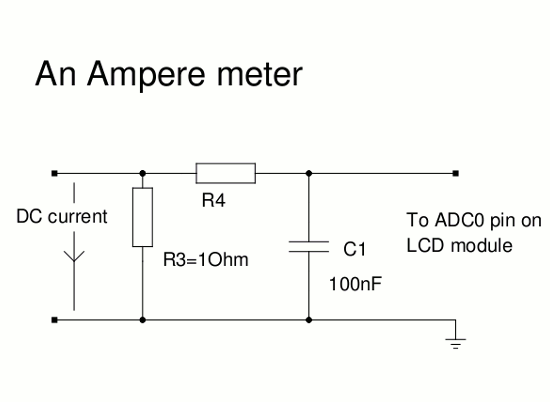The required setup for current measurements

The resistor R4 (anything between 10K and 100K) is needed to have an effective noise filter and to protect the microcontroller from high voltages in case of an accidental current spike through the 1 Ohm resistor.

The theoretical approach to determine the constants D, M, A, P would be via the following formula:
```Display_value= I*1Ohm * 4092/1.1V * M / D + A
```
I suggest however to use the more practical approach of reading out the raw digital to analog converter values at a given current and then set those constants (D, M, A, P) since it's faster and your system is calibrated in just one iteration. P=0 since we don't show any decimal point. A=0 since we do not need any offset from zero.

At a current of 1A we see the ADC reporting 3700.

We would like to display 1A as 1000mA (display should show 1000).

1000= D/M * 3700

A possible solution would be D=100 and M=370, A=0 and P=0

## FAQ part

Can I measure only analog signals?

The display module comes loaded with software that allows you to perform analog measurement (configurable DVM module software) but you can overwrite it and load your own software. You can use it as a digital clock, a counter, read digital sensors, ...

Can I get the display to show any number of digits behind the decimal point?

The display has only 8 characters so the max. is 6 digits behind the decimal point but you should not use a resolution that is higher than the accuracy of the system. The ADC produces 4092 discreet points. If you adjust the measurement range via a voltage divider such that the max. expected input corresponds 1.1V at the ADC then you can estimate the max physical resolution as: Max input voltage divided by 4092. E.g if you measure 0-10V then your theoretical max. resolution (one ADC step) is 10V/4092=0.0024V.

## References

Technical specifications:
Power: internally 5V DC, can be powered externally with 6.5-30V DC, consumes about 0.05A
Display hight: 5mm, this is the size of the actual characters on the display.
Size of the board: 5.25cm X 7.25cm (2.07x2.8 inch)

 <--, tuxgraphics Home Go to the index of this section